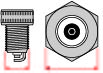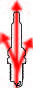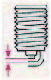# Spark Plug Nickel Standard

## DENSO IDENTIFICATION

 DENSO Identification for Standard Nickel Plug

 W 16 E X R -U 11Thread Diameter & Hexagon Size Heat Range Thread Length Electrode Design (Spark Position) Resistor Gap Configuration Spark Gap K = 14 x 16.0 Q = 14 x 16.0 W = 14 x 20.6 U = 10 x 16.0 X = 12 x 18.0 XU = 12 x 16.0 M = 18 x 25.4 L = 18 x 22.2 X = 12 x 18.0 T = 14 x 16.0 Y = 8 x 13.0 9 14 16 20 22 24 27 29 31 32 34 35 A = 19.0 B = 19.0 C = 19.0 D = 19.0 E = 19.0 F =12.7 FE = 19.0 G = 19.0 H = 26.5 L = 11.2 M = 8.6 N = 17.5 V = 25.0 B = 3 Ground Electrodes D = 4 Ground Electrodes K = 1mm Projected M = Compact Type P = 1.5mm Projected S = 0mm Projected T = Double Ground Electrodes X = 2.5mm Projected R = With Resistor None = Without Resistor C = Cut-Back Ground Electrode M =  Increased Ground Electrode Size N = For Yamaha & Kawasaki P = Double Platinum U = U-Groove US = Star-Shaped Center Electrode S = Semi-Surface Discharge Z = Taper Cut 5 = 0.5mm 8 = 0.8mm 9 = 0.9mm 10 = 1.0mm 13 = 1.3mm 14 = 1.4mm 15 = 1.5mm 20 = 2.0mm